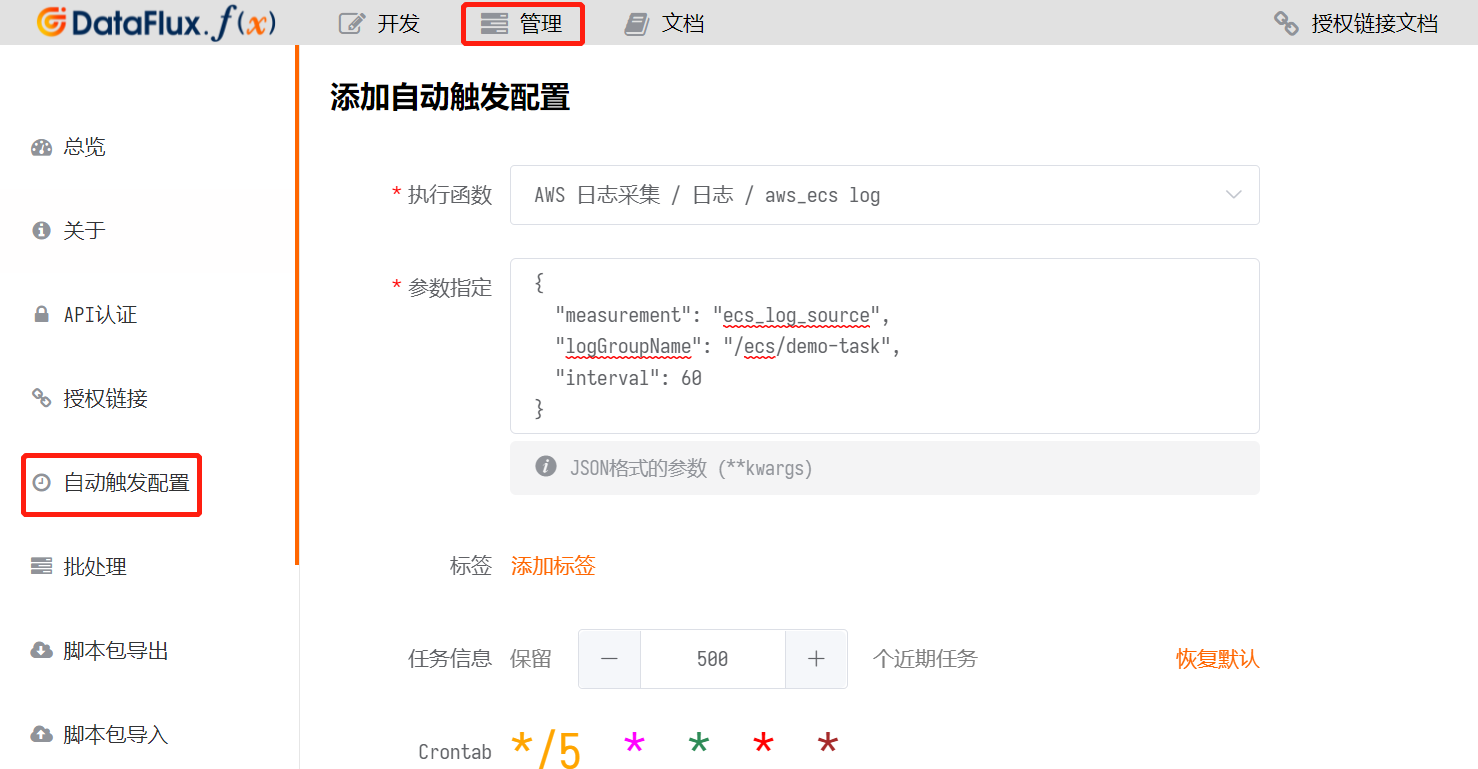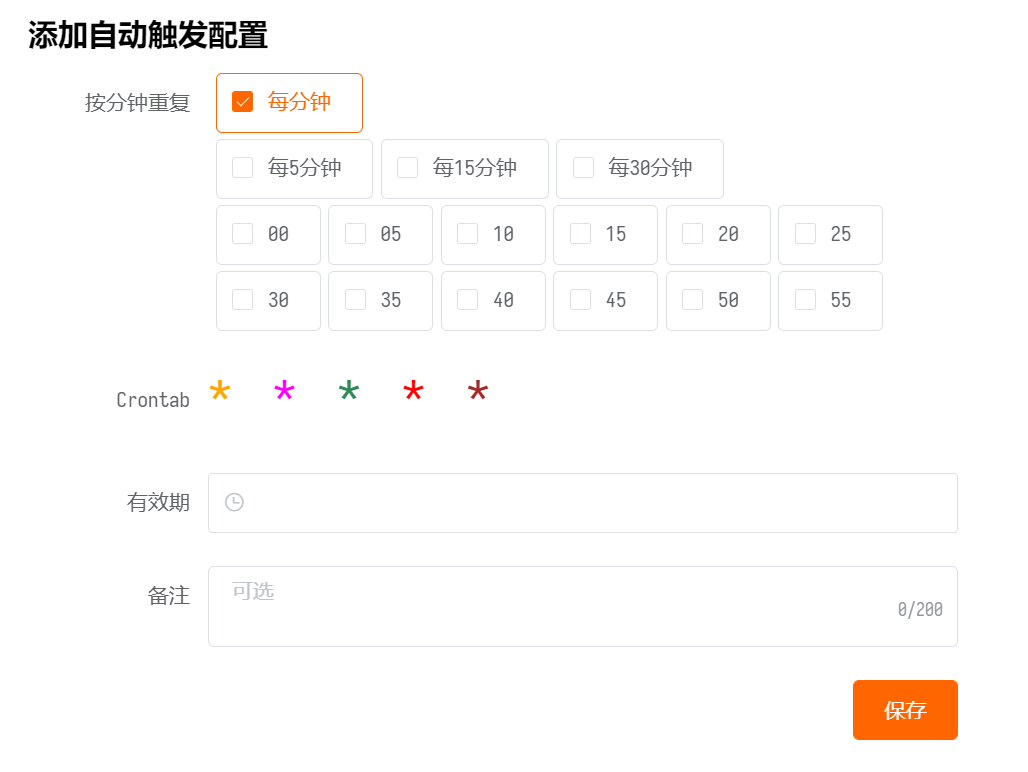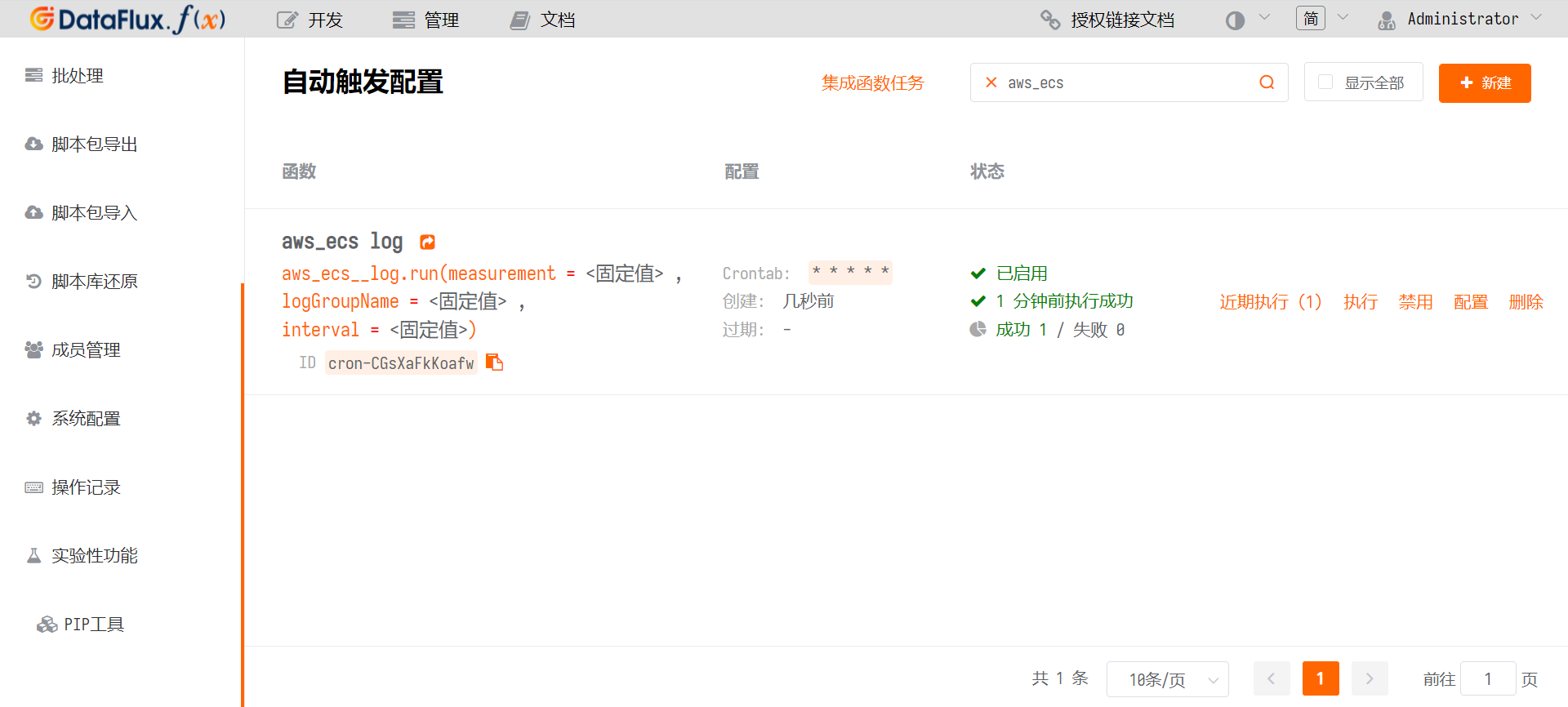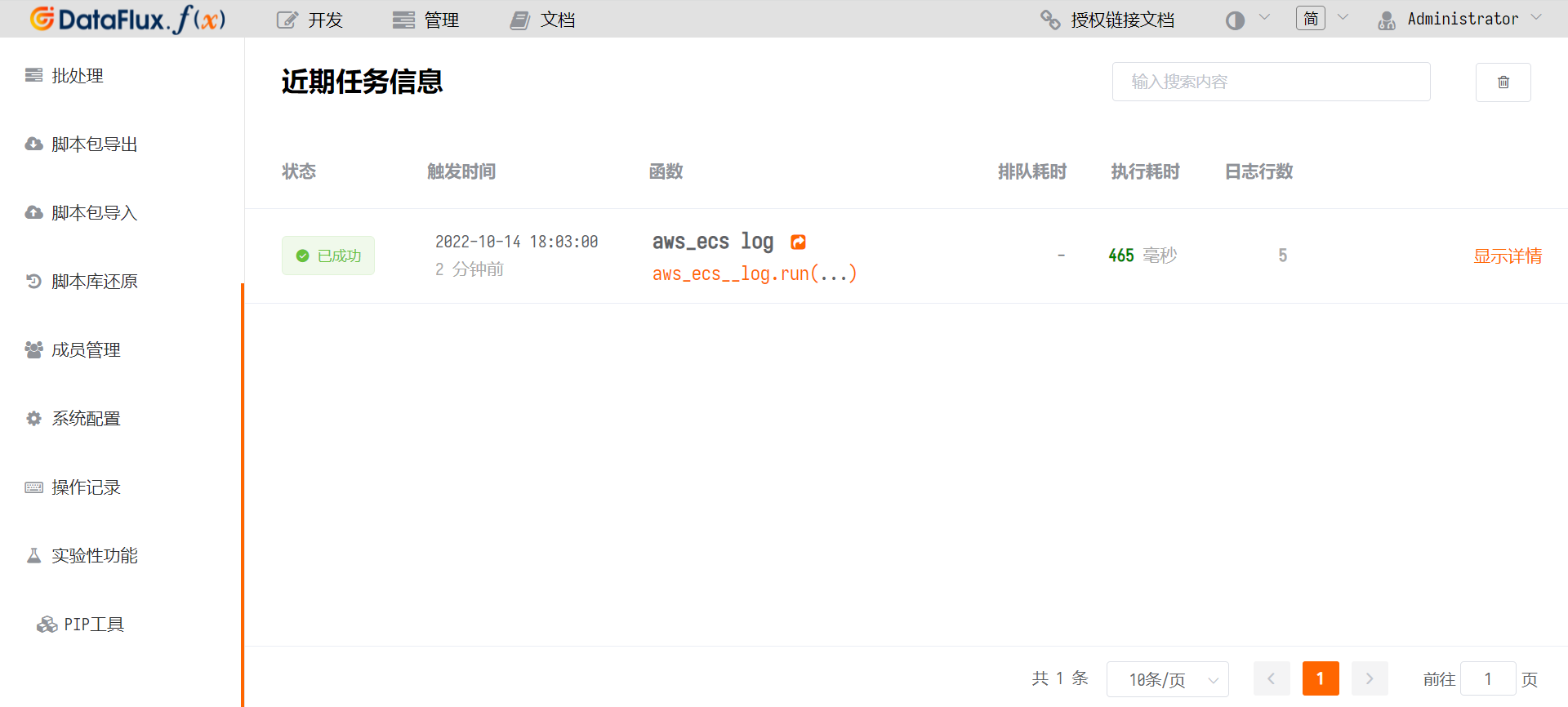# 观测云采集 Amazon ECS 日志¶

## 简介¶

Amazon Elastic Container Service (Amazon ECS) 是一项高度可扩展的快速容器管理服务，可以使用它轻松运行、停止和管理群集上的容器。这些容器可以运行在自己的 EC2 服务器上，也可以运行在由 AWS Fargate 托管的无服务器基础设施。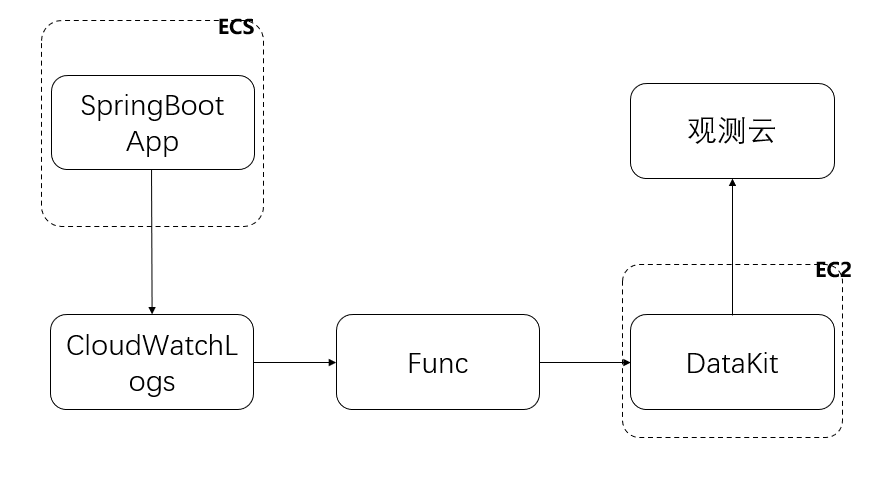## 前置条件¶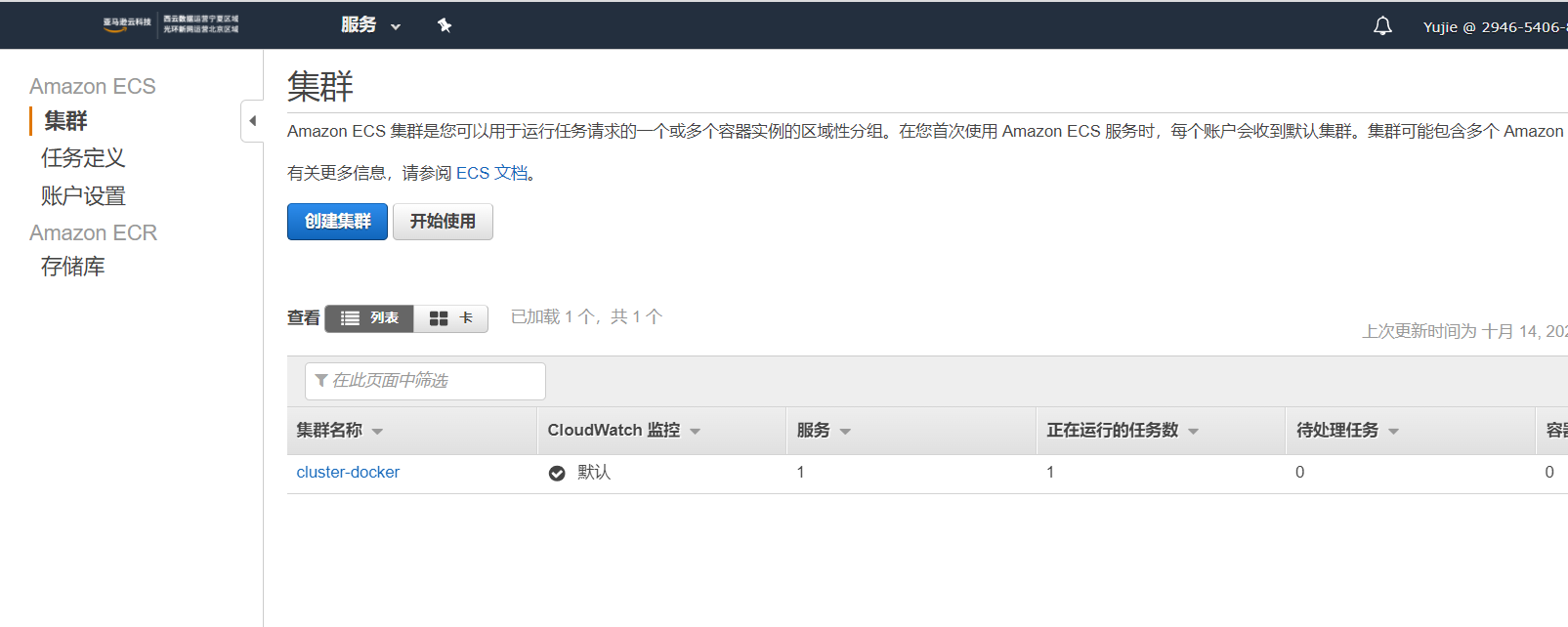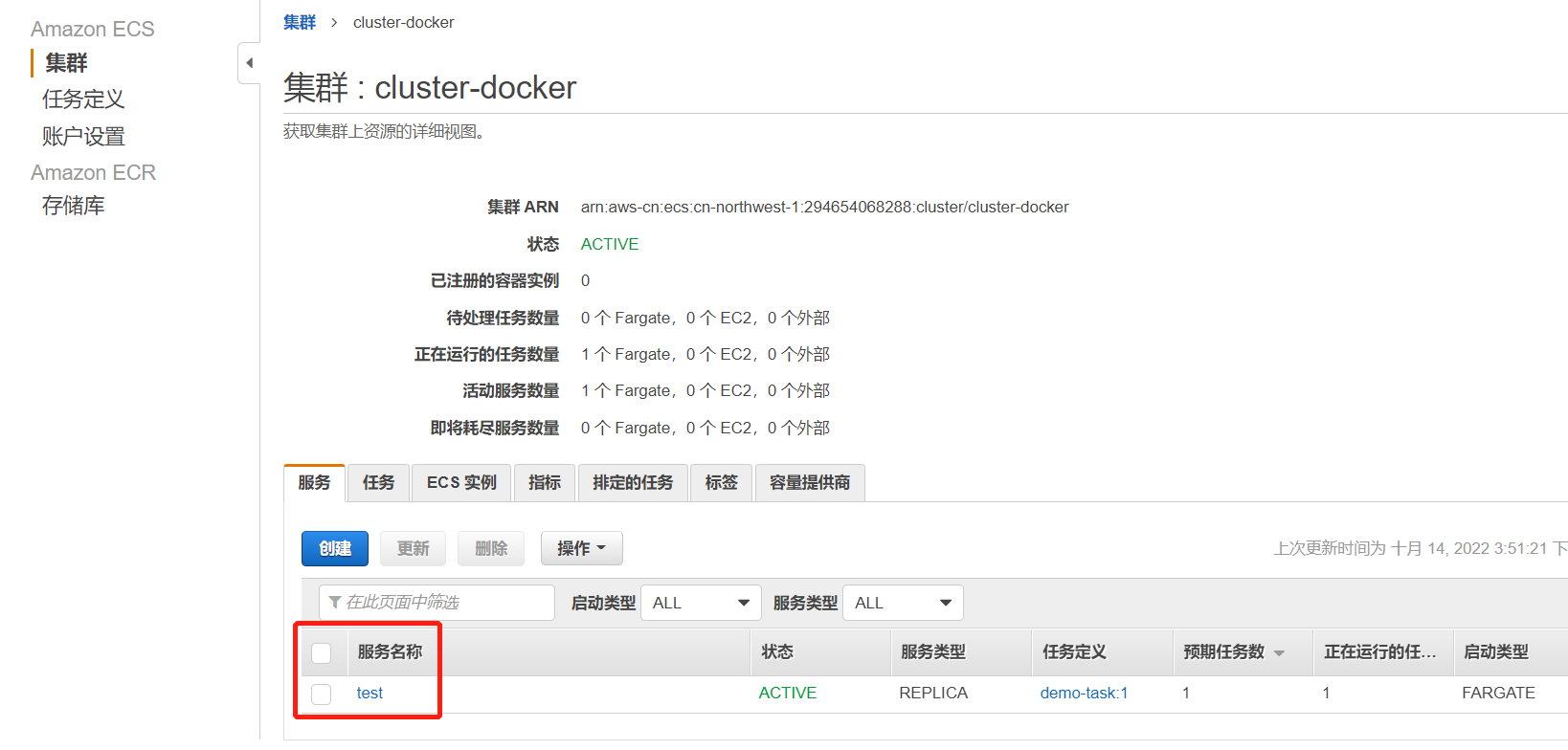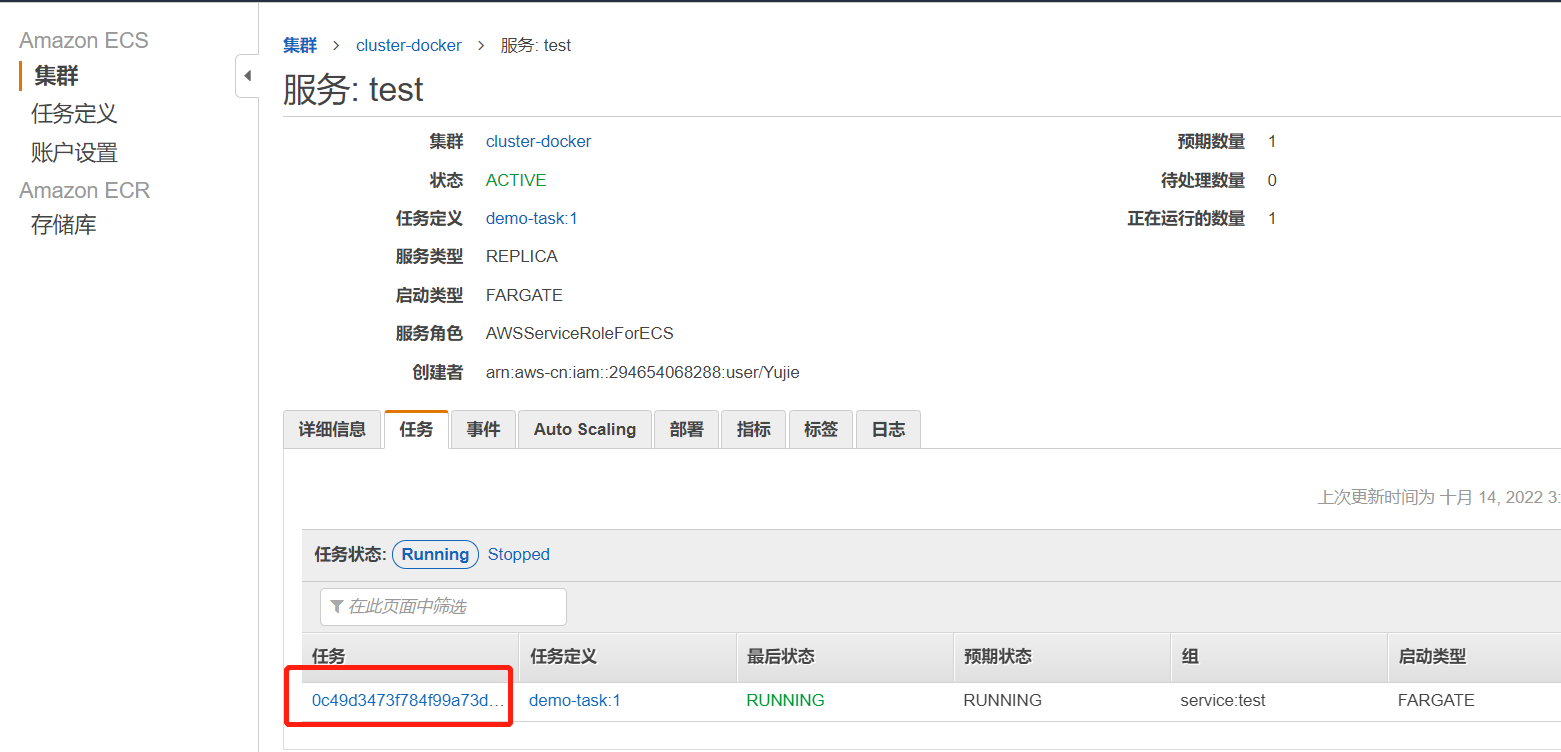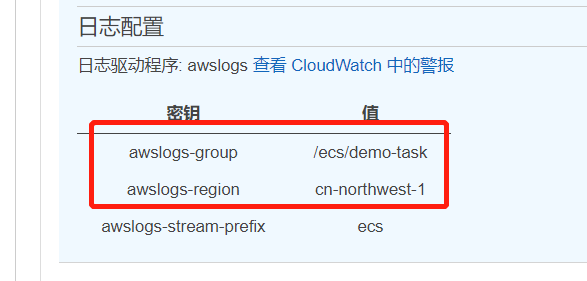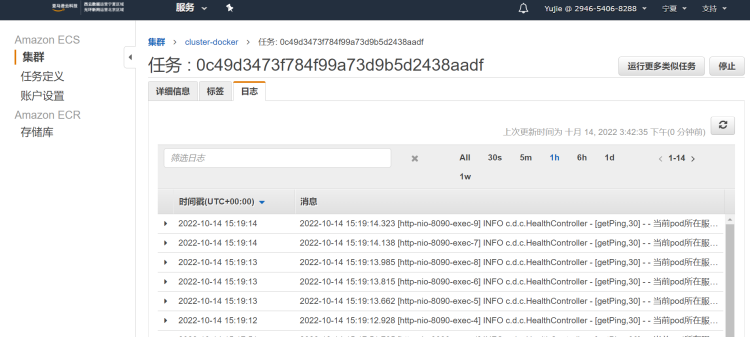## 操作步骤¶

Attention

### 步骤 1 AWS 配置¶

#### 1.2 设置 AWS 用户权限¶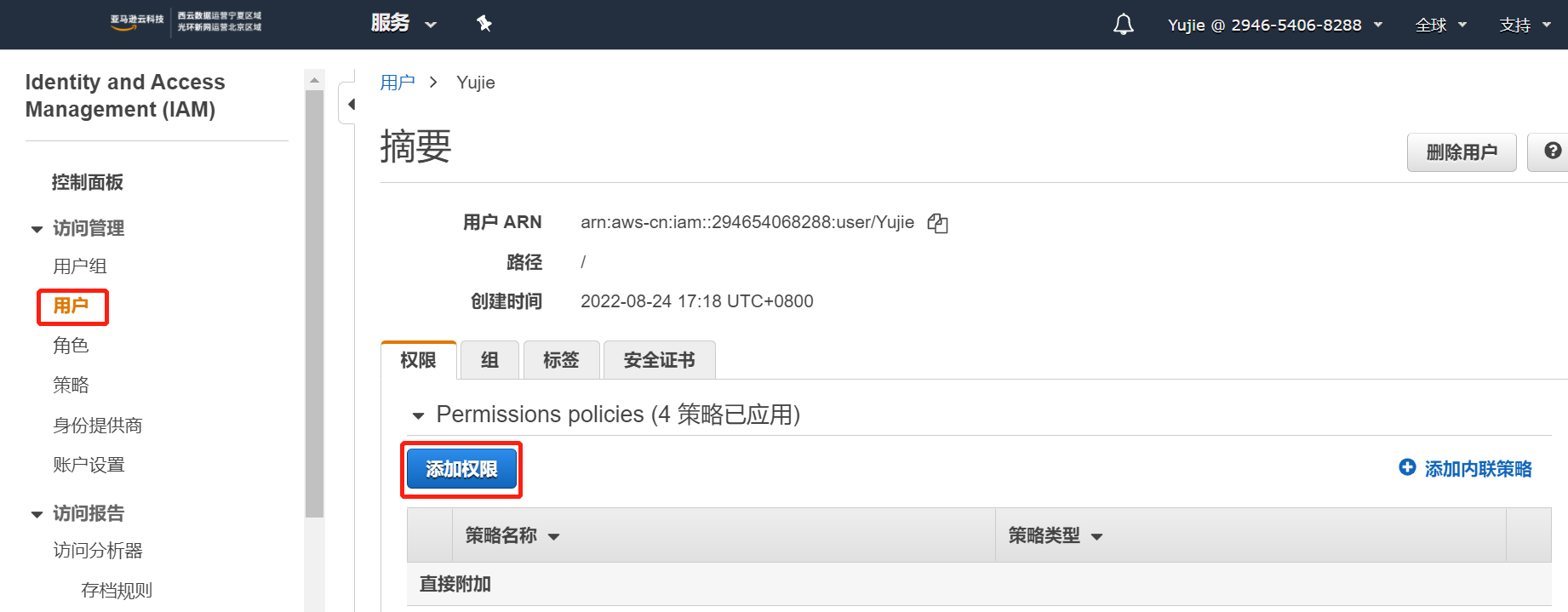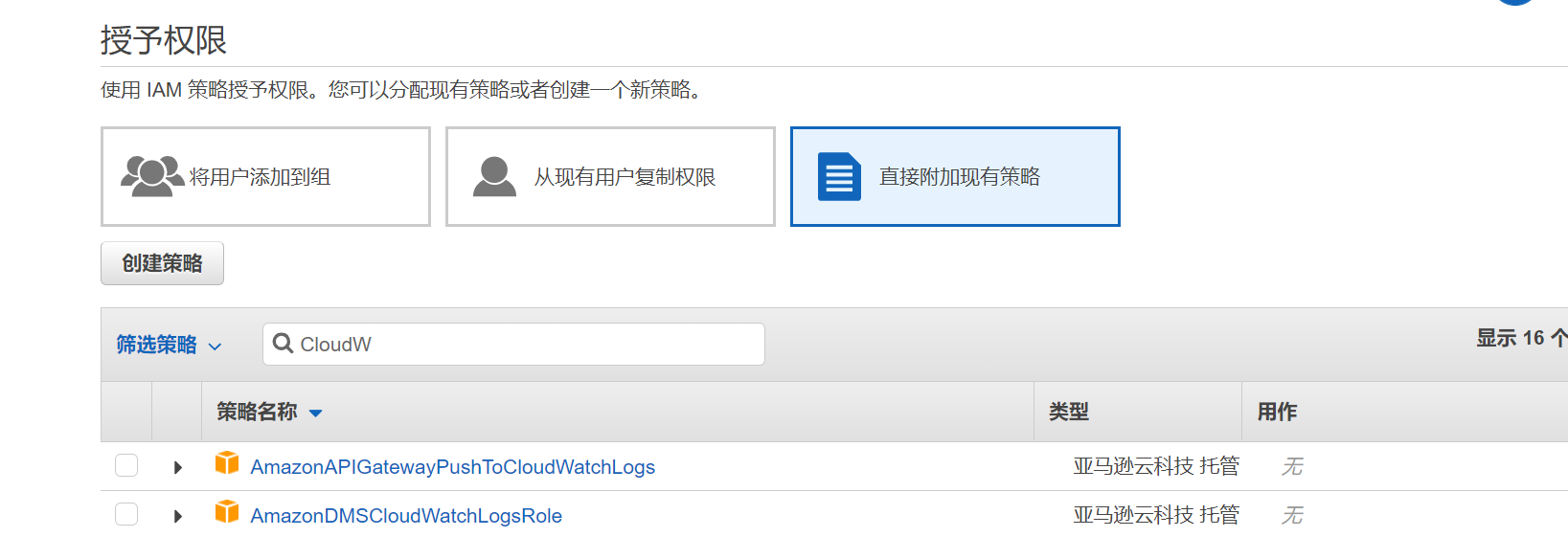### 步骤 2 Func 配置¶

#### 2.1 配置环境变量¶

• `AWS_LOG_KEY` 值对应步骤 1.1 中 AWS 用户的 `Access key ID`
• `AWS_LOG_SECRET_ACCESS_KEY` 值对应步骤 1.1中 AWS 用户的 `Secret access key`
• `AWS_REGION_NAME` 值对应 AWS 用户所在的 `REGION`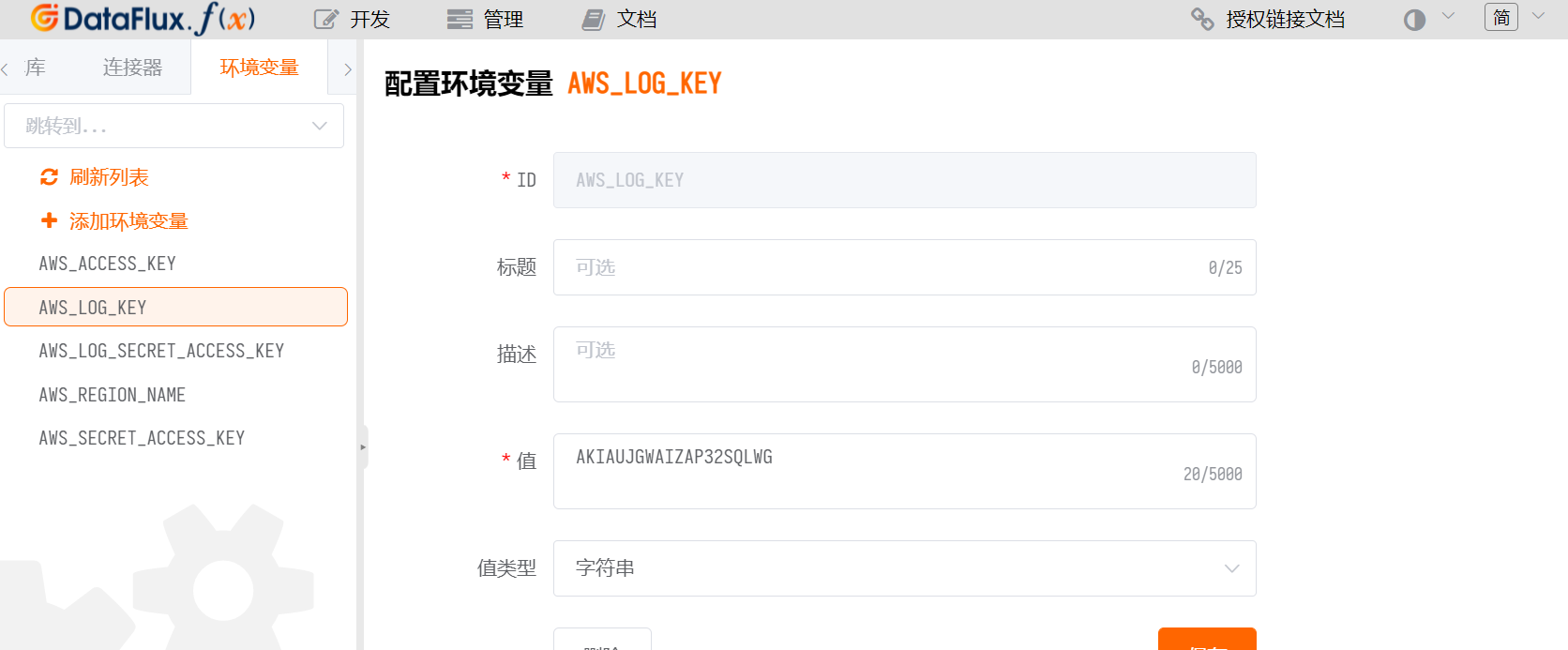#### 2.2 配置连接器¶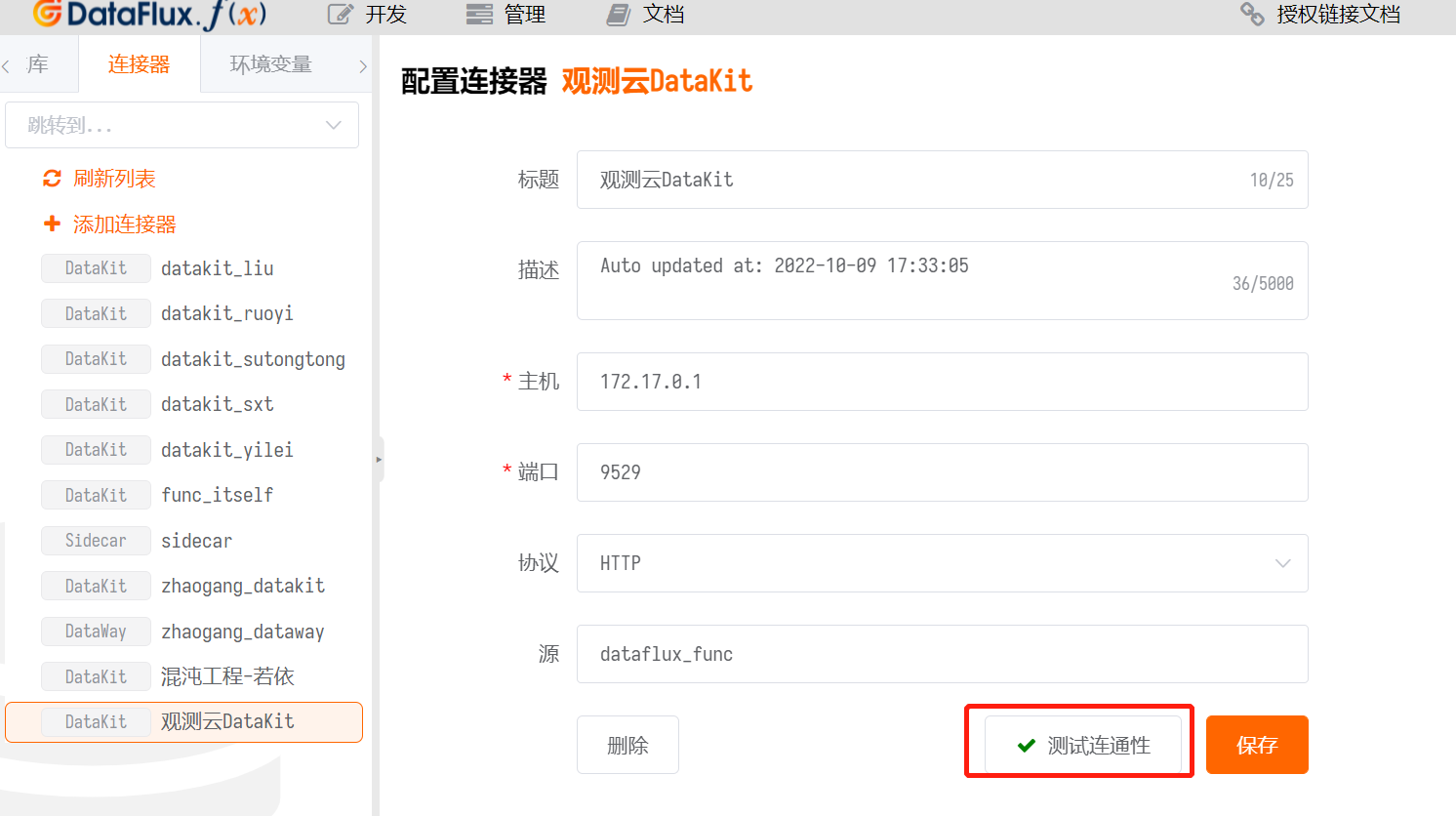#### 2.3 PIP 工具配置¶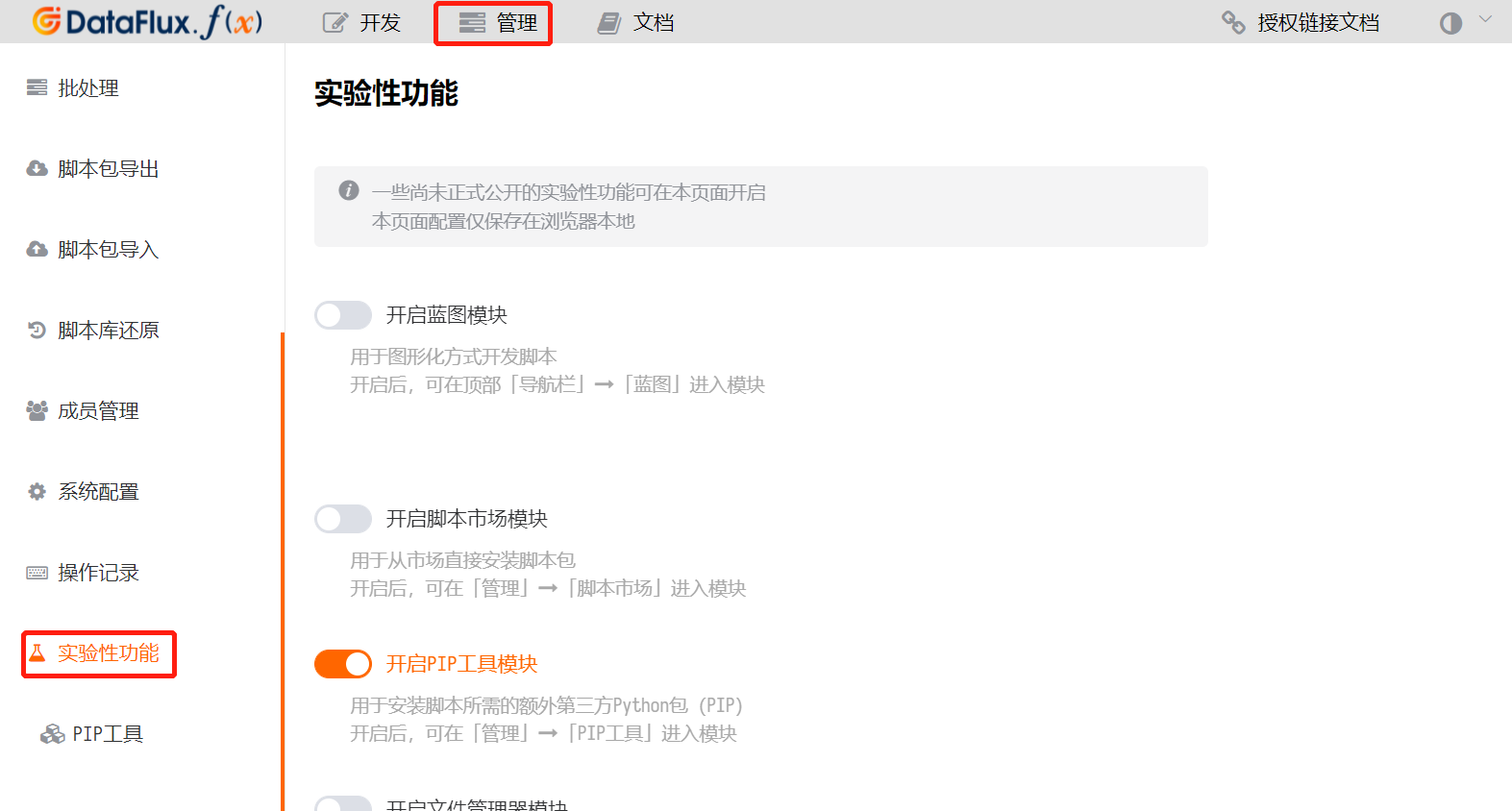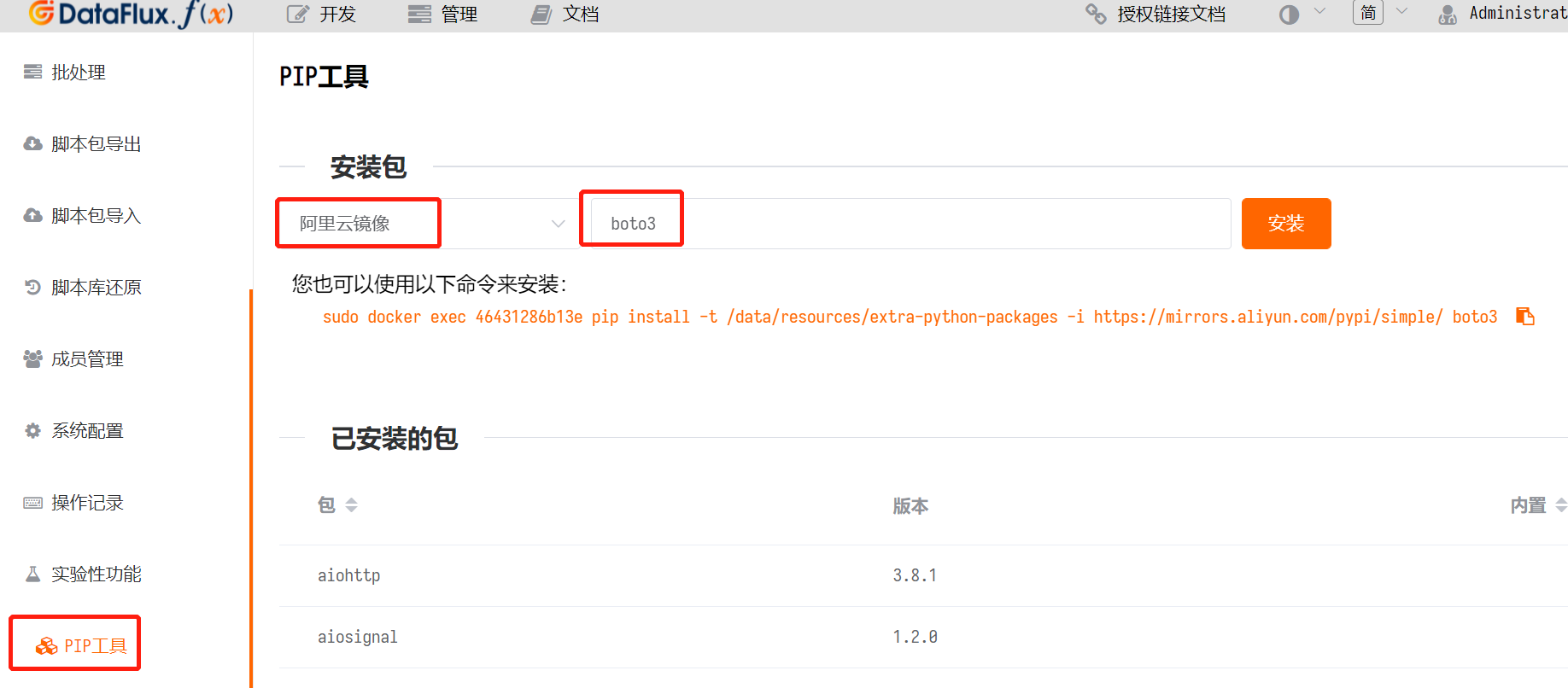#### 2.4 脚本库¶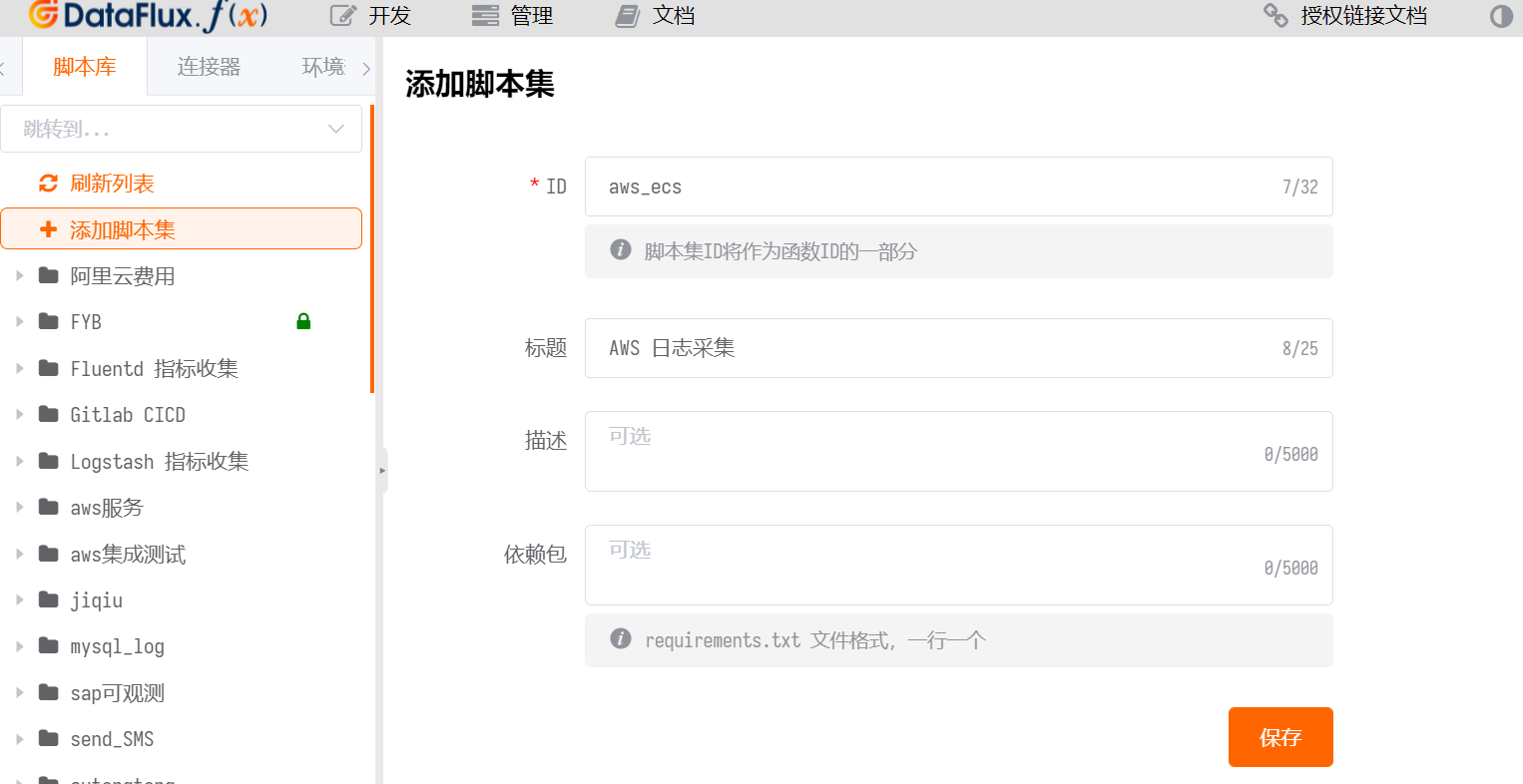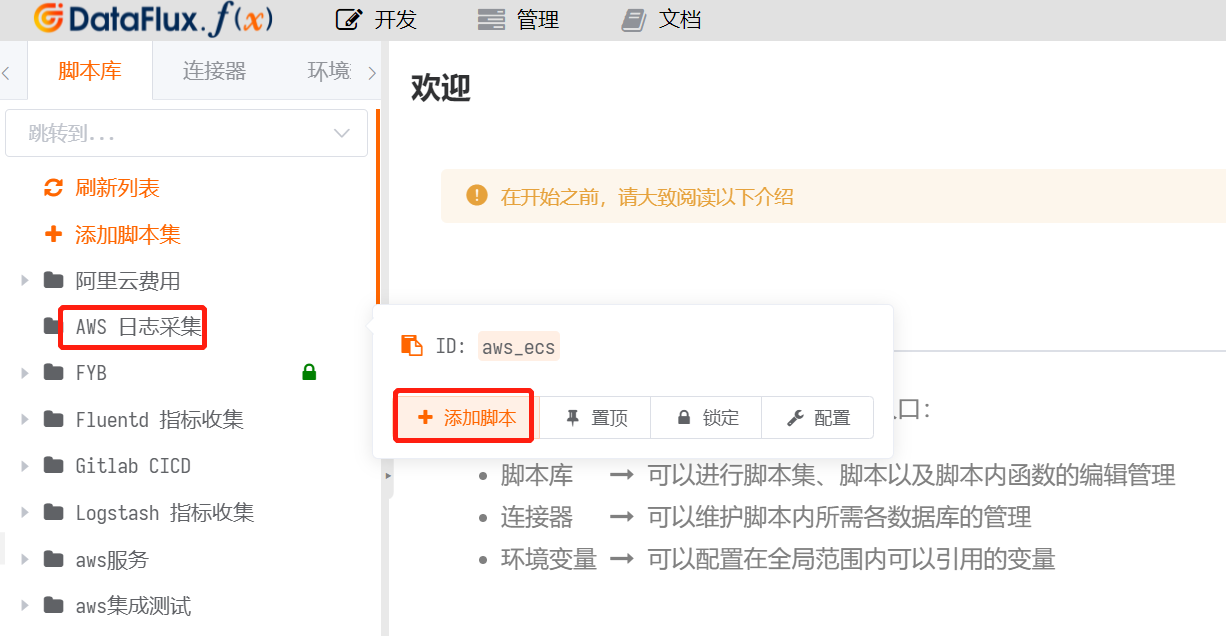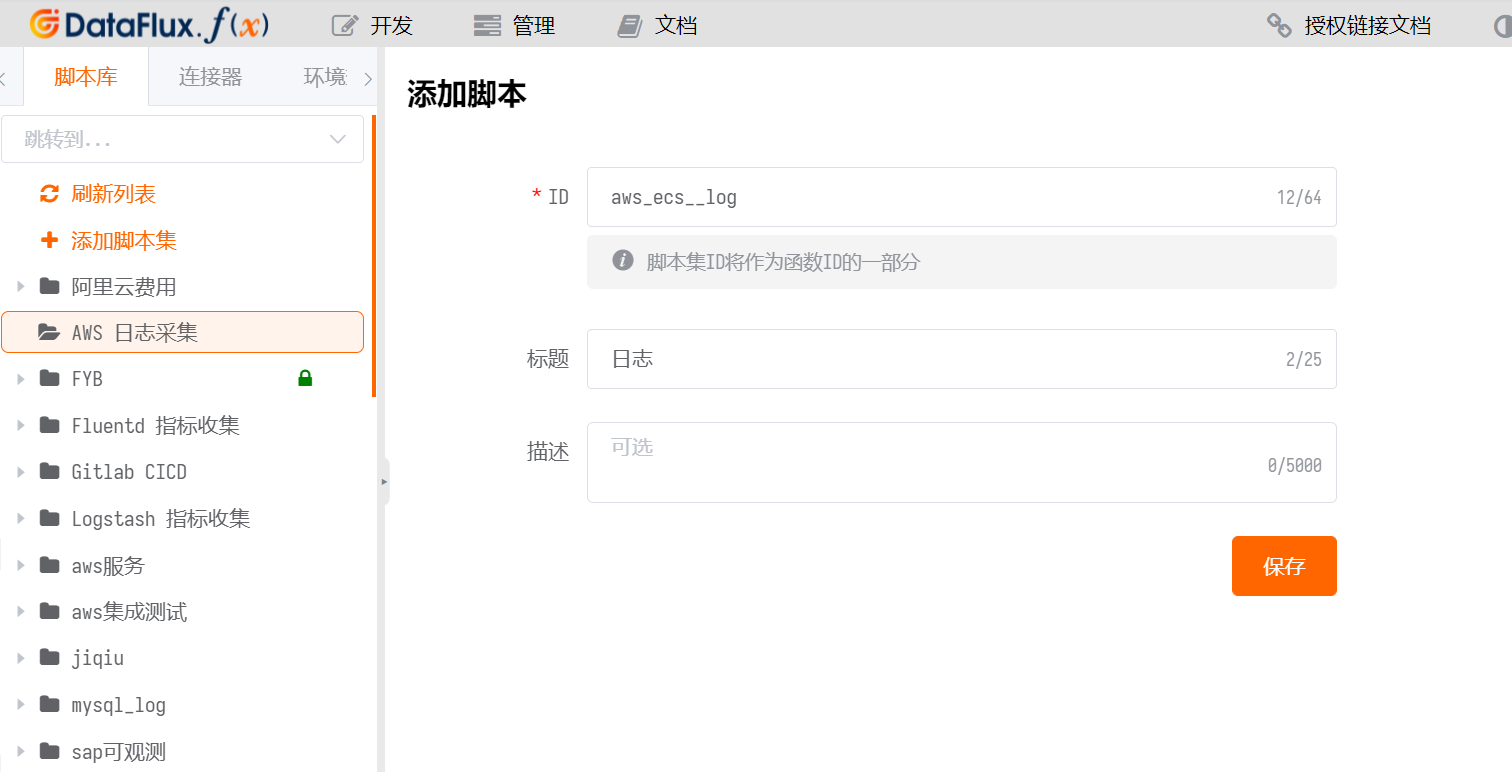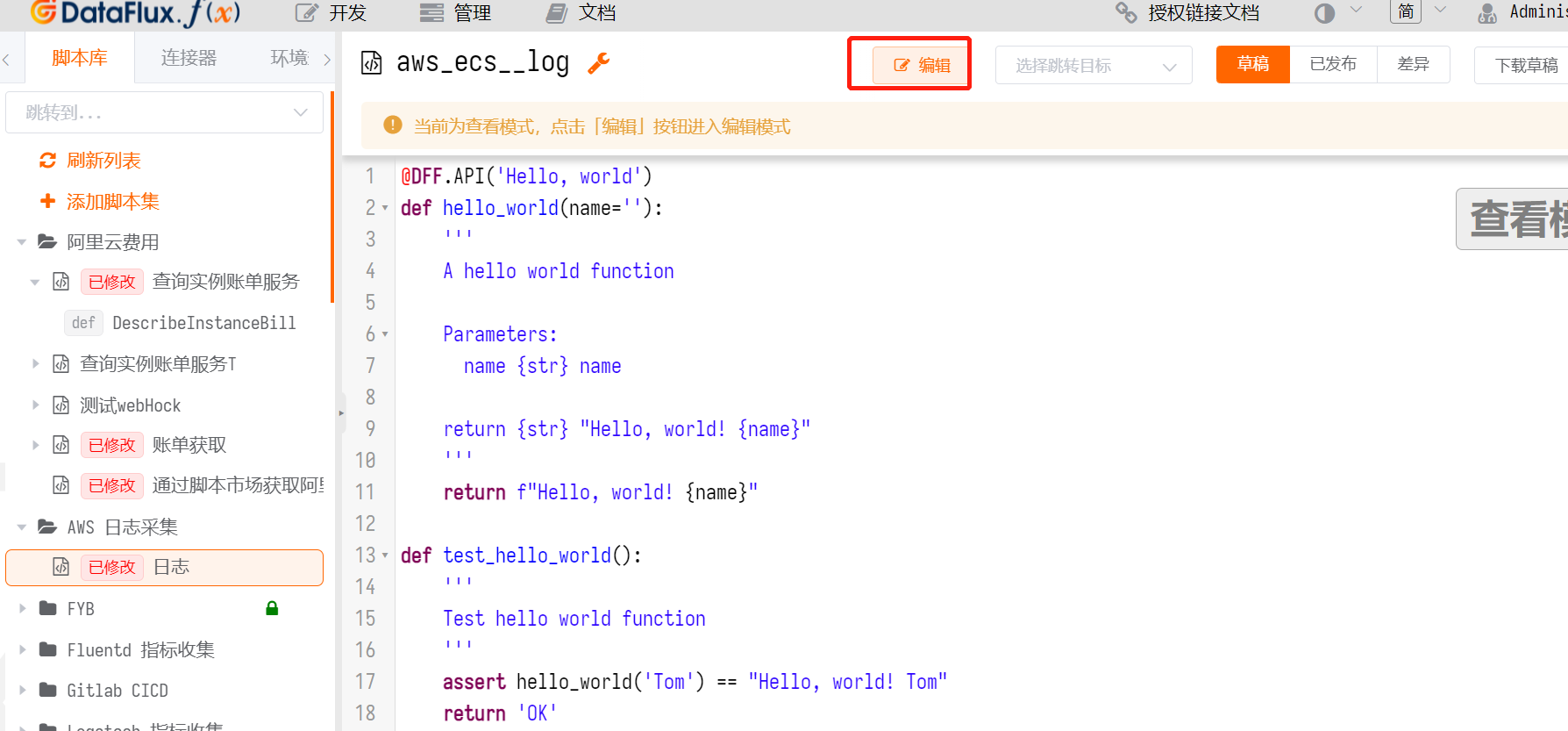``````    import boto3
import json
import time
scope_id='ecs_log'

@DFF.API('aws_ecs log', timeout=500, api_timeout=180)
def run(measurement, logGroupName, interval):
print(measurement, logGroupName, interval)
get_log_data(measurement, logGroupName, interval)
# if data is not None:
#     push_log(data)
# else:
#     print("None")

def get_cron_time(interval, measurement):
cache = DFF.CACHE.get('last_time_%s' %measurement,scope=scope_id)
if cache == None:
currentTime = int(round(time.time() * 1000))
startTime = currentTime - int(interval) * 1000
endTime = currentTime
else:
currentTime = int(round(time.time() * 1000))
if currentTime - int(cache) > 10 * 60 * 1000:
startTime = currentTime - int(interval) * 1000
endTime = currentTime
else:
startTime = int(cache) + 1
endTime = currentTime
print(startTime, endTime)
return  startTime, endTime

def get_log_data(measurement, logGroupName, interval):
logTime = get_cron_time(interval, measurement)
startTime = logTime
endTime = logTime
isPush = False
client = boto3.client(
'logs',
aws_access_key_id=DFF.ENV('AWS_LOG_KEY'),
aws_secret_access_key=DFF.ENV('AWS_LOG_SECRET_ACCESS_KEY'),
region_name=DFF.ENV('AWS_REGION_NAME')
)# print(client.meta.config)
try:
nextToken = 'frist'
logData = []
while nextToken != '':
if nextToken == 'frist':
nextToken = ''
response = client.filter_log_events(
logGroupName=logGroupName,
startTime=startTime,
endTime=endTime,
limit=1000,
#filterPattern="?ERROR ?WARN ?error ?warn",
interleaved=False
)
else:
response = client.filter_log_events(
logGroupName=logGroupName,
startTime=startTime,
endTime=endTime,
nextToken=nextToken,
limit=1000,
#filterPattern="?ERROR ?WARN ?error ?warn",
interleaved=False
)
try:
if len(response['events']) > 0:
data = []
lastTimeList = []
for i in response['events']:
# print("hii", i['logStreamName'])
log = {
'measurement': measurement,
'tags': {
'logGroupName': logGroupName,
'logStreamName': i['logStreamName'],
'host': '127.0.0.1'
},
'fields': {
'message': i['message'],
'time': i['timestamp']
}
}
data.append(log)
lastTimeList.append(i['timestamp'])
push_log(data)
print("max %s"  % max(lastTimeList))
DFF.CACHE.set('last_time_%s' % measurement, max(lastTimeList), scope=scope_id, expire=None)
isPush = True
else:
DFF.CACHE.set('last_time_%s' % measurement, endTime , scope=scope_id, expire=None)
nextToken = response['nextToken']
except:
nextToken = ''
except Exception as  e:
print('Error: %s' % e )
return None
if not isPush:
DFF.CACHE.set('last_time_%s' % measurement, endTime , scope=scope_id, expire=None)

def push_log(data):
datakit = DFF.SRC('datakit')
status_code, result = datakit.write_logging_many(data=data)
if status_code == 200:
print("total %d"  % len(data))
print(status_code, result)
``````
Attention
• 上述内容第4行的 `ecs_log` ，需要确保同一个 Func 中唯一，可以改成其它字母。
• 第6行的 `awc_ecs` 即是刚才添加的脚本集 ID
• 第 40、41、42 行中的 `AWS_LOG_KEY``AWS_LOG_SECRET_ACCESS_KEY``AWS_REGION_NAME` 对应步骤 2.1 中的环境变量名，如果环境变量名变了，需要做对应修改。

#### 2.5 测试脚本¶

• `measurement` 的值输入 `ecs_log_source`，这个值对应观测云日志中的日志来源；
• `logGroupName` 的值对应前置条件的日志配置中查到的 `awslogs-group`;
• `interval` 的值对应采集频率，示例这里是 60 秒。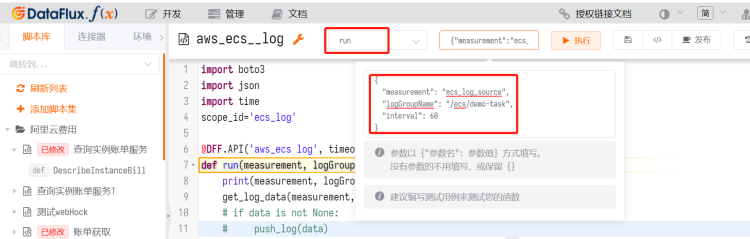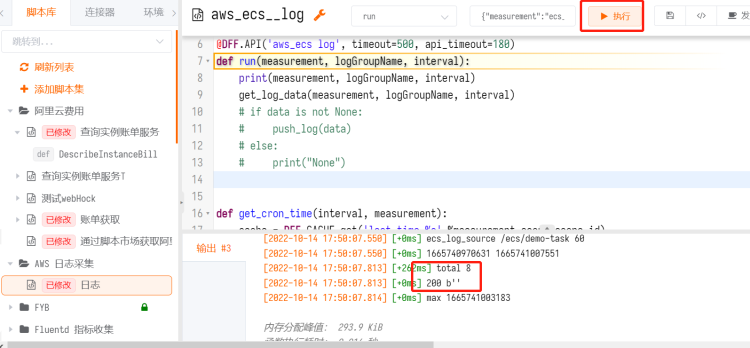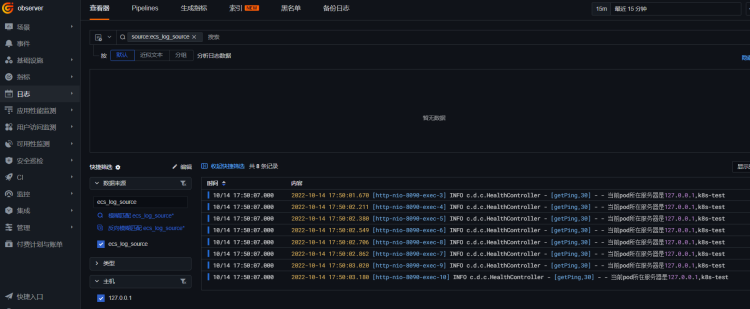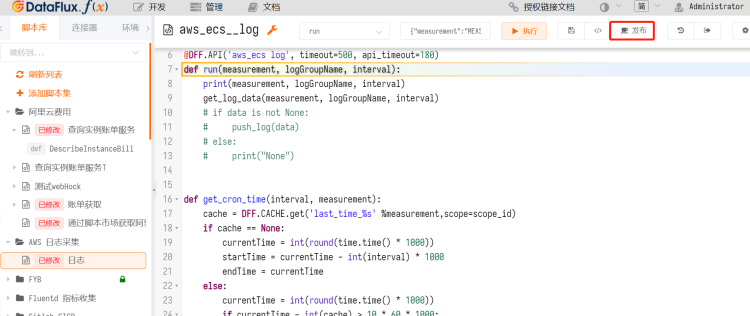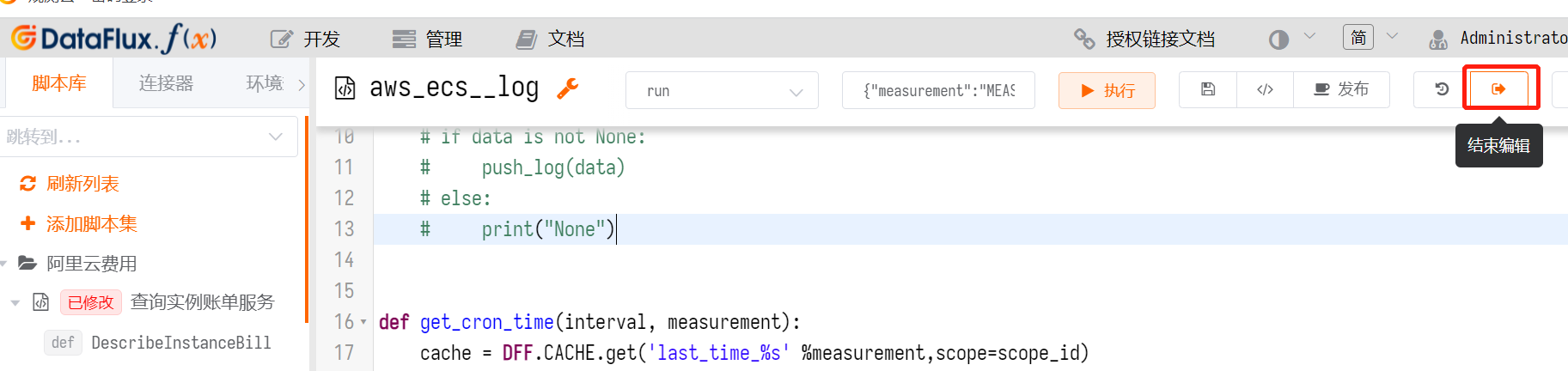#### 2.6 自动采集日志¶

``````{
"measurement": "ecs_log_source",
"interval": 60
}
``````